# Fraction calculator v3.0.0 (paid) Apk

A handy fraction calculator with steps, that helps you add fractions, subtract fractions, multiply fractions, and divide fractions. This...

A handy fraction calculator with steps, that helps you add fractions, subtract fractions, multiply fractions, and divide fractions.
This fractions calculator performs operations on proper and improper fractions, mixed numbers (mixed fractions), and whole numbers.
Fraction calculator can be used to calculate two and three fractions.
To solve fractions, select the number of fractions, type in fractions, mixed numbers, or whole numbers in the provided fields, select the operation and click “Calculate”. You can enter negative fractions by putting a minus sign before the whole part.
Fraction calculator lets you quickly solve homework problems or check results.
Features:
Fraction solver for two and three fractions.
Solves several types of problems, including addition, subtraction, multiplication, and division, with 2 or 3 fractions.
Operations on proper fractions, improper fractions, mixed numbers and whole numbers.
Supports negative values.
Adding and subtracting fractions with like denominators.
Adding and subtracting fractions with unlike denominators. In case of adding and subtracting fractions with unlike denominators the fractional calculator finds the least common denominator.
Multiplying and dividing fractions.
Multiplying and dividing mixed fractions.
Shows step by step instructions.
History tape to view your recent calculations.
Back and forward buttons to check or recall recent calculations.

Fractions solver sends results and calculation history via email.
'Undo' for the Clear command.
Supports both portrait and landscape orientation.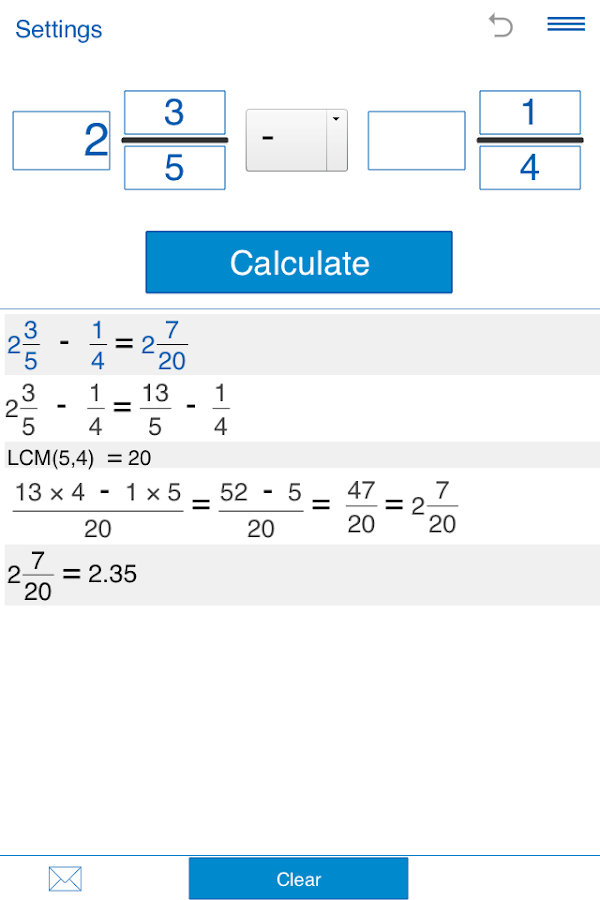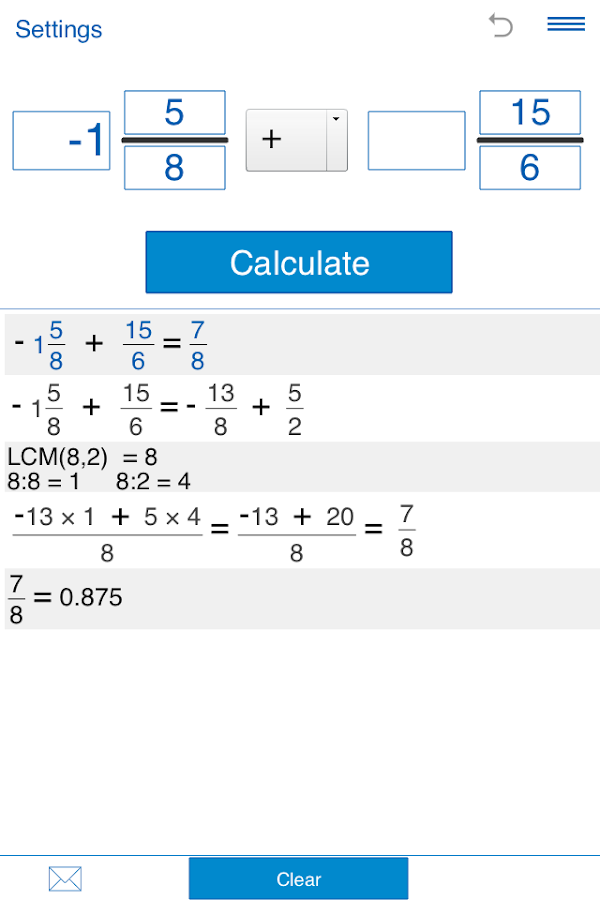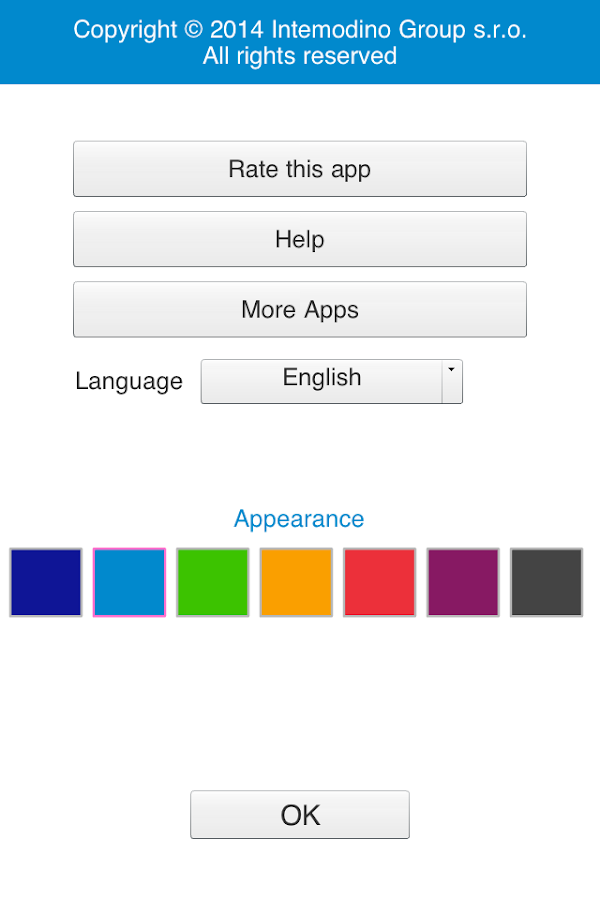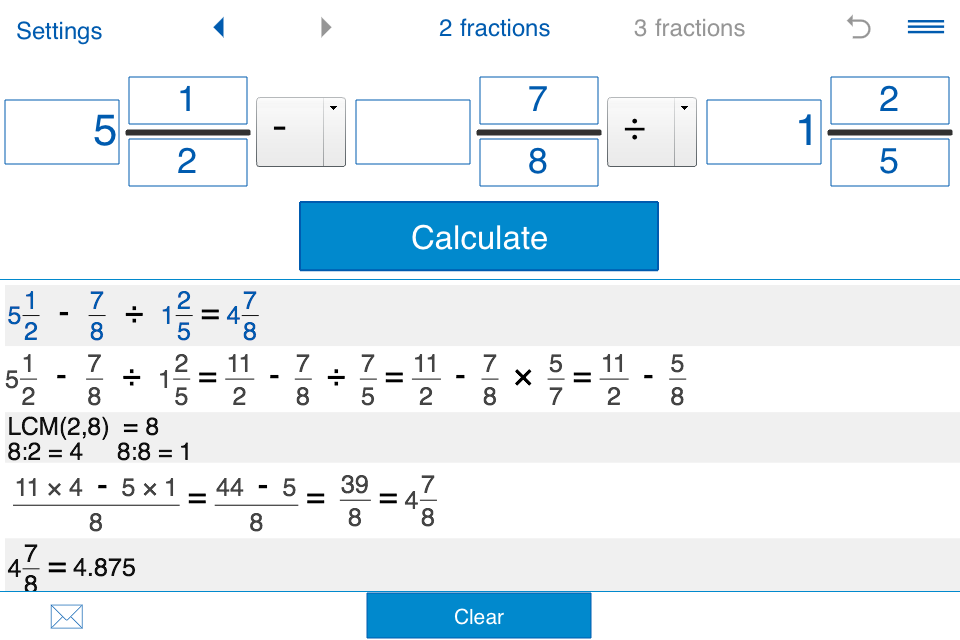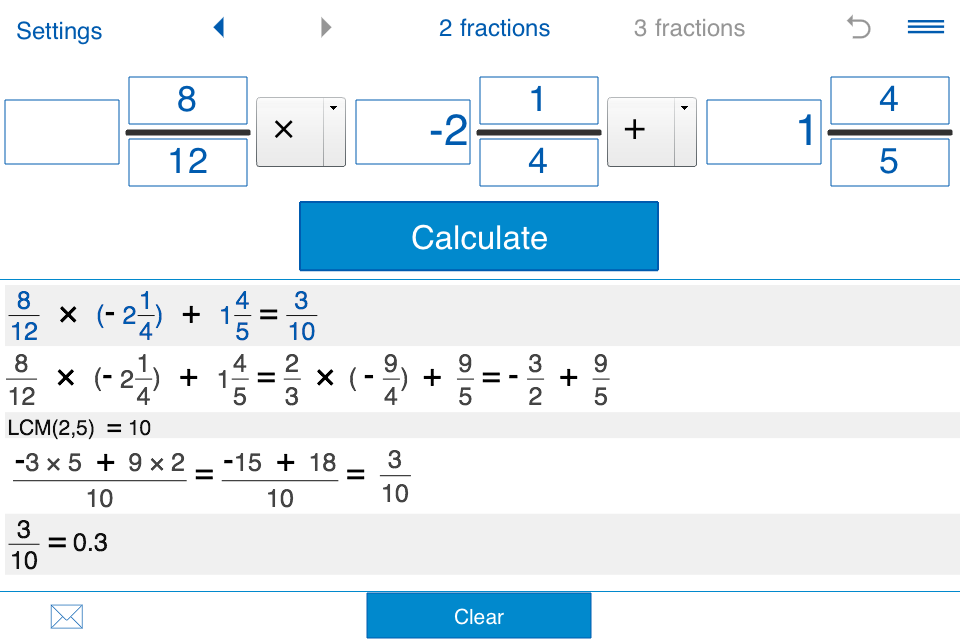# WHAT'S NEW

New interface.
General improvements.
New app icon.

Requires Android-2.2 and up